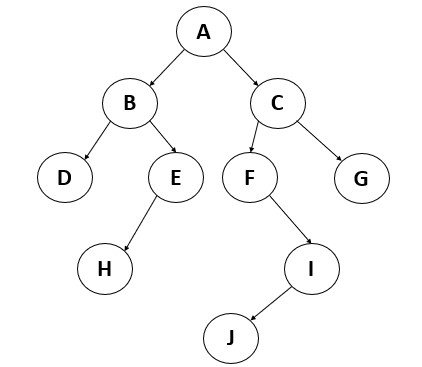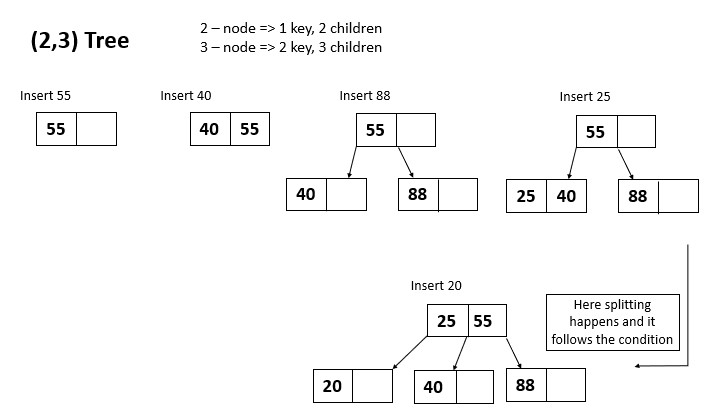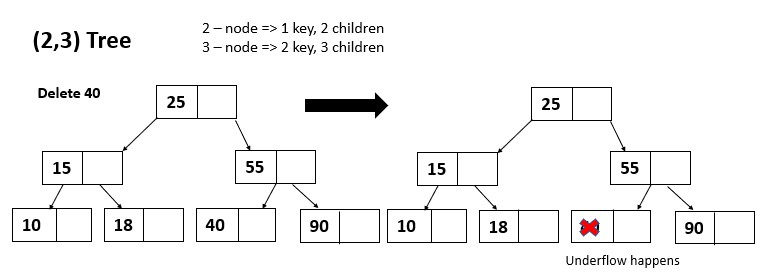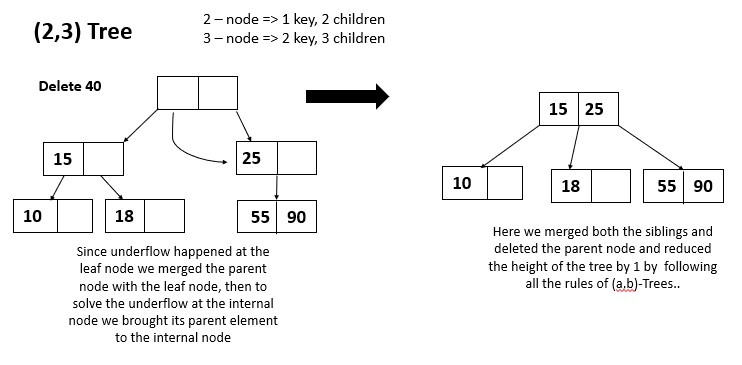×

Search anything:

# (a,b) Tree Data Structure

#### Data Structures AlgorithmsAn (a,b)-tree is a type of self-balancing tree data structure that is designed to maintain a balance between the height of the tree and the number of elements stored in the tree. In short we can say that (a,b)-tree is a generalization of a B-tree. Now lets get to know it in depth.

• Prerequisites
• Tree Data Structure
• Self-Balancing Tree
• What Are (a,b)-Trees
• Search Operation
• Insertion Operation
• Deletion Operation
• Applications

## Prerequisites

These are some of the things we should know before we get into (a,b)-Tree.

### Tree Data Structure

A tree is a non-linear data structure which consists of nodes arranged in a hierarchy. Each node has a value and a list of references to other nodes (its children).
The nodes with children are called internal nodes and the nodes without children are called the leaf nodes. The top most node is called the root or root node.

Some common tree terminologies:

• The depth of a node is the number of edges from the root to the node.
• The height of a node is the number of edges from the node to the deepest leaf.
• The height of the tree is the height of the root.
• The ancestor of a node is a parent, grandparent, great-grandparent, and so on.
• The descendant of a node is a child, grandchild, great-grandchild, and so on.

Eg: The below image is of a tree and following that are the terminologies related to it.Here the depth of the node I is 3 (AC, CF, FI)
Here the height of the node I is 1 (IJ)
Here the height of the tree is 4.
Here the ancestor of the node F is C and A.
Here the descendant of node F is I and J.

There are many different types of trees, including:

• Binary trees: Each node has at most two children.
• Binary search trees: A binary tree where the value of each left child is less than its parent, and the value of each right child is greater than its parent.
• Tries: A tree where the edges represent sequences of characters, and the nodes represent common prefixes.
• Heaps: A tree where the value of each node is greater than or equal to its children (for a max heap) or less than or equal to its children (for a min heap).

### Self-Balancing Tree

Self-balancing trees are a type of tree data structure that automatically keep their height as small as possible by rearranging the tree when nodes are added or removed. This is done to keep the tree balanced, which has several benefits.

Benefits of self-balancing trees include:

• They can keep their height small, which means that search, insertion, and deletion operations can be done in logarithmic time (O(log n)). This is faster than an unbalanced tree, which can have a height of O(n).
• They can automatically adapt to changing data patterns. For example, if a tree is used to store a sorted list of numbers that are frequently accessed in a certain order, a self-balancing tree can rearrange itself to make those accesses faster.

There are many different types of self-balancing trees, including AVL trees, red-black trees, and splay trees. Each of these types uses a different method to maintain balance, such as rotations, recoloring of nodes etc.

## What Are (a,b)-Trees

(a,b)-trees are a type of balanced tree data structure that are used to store and retrieve ordered data.They are similar to other balanced tree structures such as B-Trees, red-black trees and AVL trees, but they have a more relaxed balance condition that allows them to have better performance in some cases.

In an (a,b)-tree, each node can have between a and b children, where a and b are positive integers. The root node is required to have at least two children unless it is the only node in the tree, in which case it can have one child or no children. All other nodes must have between a and b children.

The main advantage of (a,b)-trees is that they have good performance for both search and insert/delete operations. Search time is logarithmic, like other balanced tree structures, and insert/delete time is O (log n + k), where k is the number of nodes that are split or merged as a result of the operation. This is faster than some other balanced trees, which can take longer for insert/delete operations because they have a stricter balance condition.

## Search Operation

In an (a,b)-tree, the search operation works by starting at the root node and comparing the search key with the value stored at the current node. If the search key is less than the value at the current node, the search continues in the left subtree. If the search key is greater than the value at the current node, the search continues in the right subtree. This process is repeated until the search key is found or it is determined that the key is not present in the tree. If you had read my work on B+ Tree you would recall that the search operation works the same way in it too. Thats why we say an (a,b)-tree is a generalization of a B-tree.

In the best case, the search key is found in the first node that is searched, and the search operation takes a single step so O(1).

In the average case, the search key is likely to be found after searching a small number of nodes, and the search operation will take O(log n) steps.

In the worst case, the search key is not present in the tree, and the search operation will have to search every node in the tree before determining that the key is not present. In this case, the search operation will also take O(log n) steps.

The space complexity of the search operation is O(1) in all cases, because it only requires a small amount of memory to store the search key and a few pointers to traverse the tree.

## Insertion Operation

For insertion the first step will be to search for the correct position to insert the new key. If the insertion of the new key causes the leaf node to exceed the maximum number of keys allowed (b-1), the leaf node is split into two nodes and the median (middle) key is promoted to the parent node. This process is repeated up the tree until a node is reached that does not exceed the maximum number of keys allowed. If the root node is split and a new root is created, the height of the tree is increased by 1.

In the best case key is inserted in O(1) time complexity if its being inserted in the root or children of root node (where the root has only very few children). In average and in worst case it will take O(log n + k) time where n is the number of nodes and k is the number of nodes split or merged. The log n component comes in as the tree is balanced and the height of the tree is logarithmic in the number of nodes. Worst case happens when the tree is poorly balanced or if the data entered is highly ordered.

The space complexity in all cases is O(1).## Deletion Operation

For deletion operation also first we have to locate the key in the tree. If its found in the leaf node then its simply deleted from the node. If its found in an internal node, then it is replaced with its predecessor (the largest key in the left subtree) or its successor (the smallest key in the right subtree). The predecessor or successor is then deleted from its leaf node.

If the deletion of a key causes the node to have fewer than the minimum number of keys (a-1), the node is merged with one of its siblings. This process is repeated until a node is reached that has at least the minimum number of keys allowed. If the root node is merged with one of its children and the root becomes a leaf node, thee height of the tree is decreased by 1.

The time complexity of the deletion operation in an (a,b)-tree is O(log n + k) in the best, average, and worst case scenarios.

In the best case, the deletion operation takes a single step, because the key is found in a leaf node and is deleted without causing any splits or merges. If only root node is present and we need to delete it then it would take only O(1) time.

In the average case, the deletion operation may require a small number of splits or merges, and it will take O(log n + k) steps.

In the worst case, the deletion operation may require a large number of splits or merges, and it will take O(log n + k) steps. This can happen if the tree is poorly balanced or if the data being deleted is highly ordered.

The space complexity of the deletion operation is O(1) in all cases.## Applications

(a,b)-trees are a type of balanced tree data structure that are used to store and retrieve ordered data. They are commonly used in applications that require fast search and insert/delete operations on large data sets, such as:

• Database systems: (a,b)-trees are used to store and index data in databases, which allows for fast search and update operations.
• File systems: (a,b)-trees can be used to store the directory structure and file names in a file system, which allows for fast search and insertion/deletion of files.
• Text editors: (a,b)-trees can be used to store the text of a document and allow for fast insertion/deletion of characters.

(a,b)-trees are similar to other balanced tree data structures such as B-trees and AVL trees, but they have a more relaxed balance condition that allows them to have better performance in some cases.

So we have reached the end of the article at OpenGenus, so by now you would have a full idea on how an (a,b) tree works.

#### Aswin Shailajan

Aswin Shailajan is a B. Tech Computer Science student at Vellore Institute of Technology and is a SDE, Intern at OpenGenus.

(a,b) Tree Data Structure